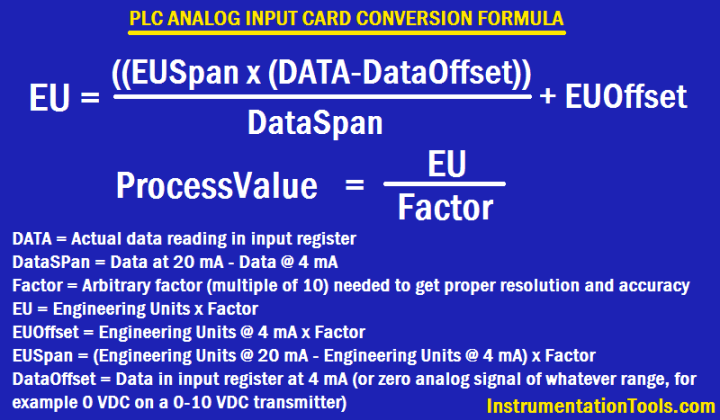For any unit conversion on any PLC, you can use four function math with the following for PLC Analog Input Conversion Formula, which assumes integer math, as follows :

## PLC Conversion FormulaFormulas:

#### ProcessValue = EU / FACTOR

DataOffset = Data in input register at 4 mA (or zero analog signal of whatever range – for example 0 VDC on a 0-10 VDC transmitter)

DataSPan = Data at 20 mA – Data @ 4 mA

FACTOR = arbitrary factor (multiple of 10) needed to get proper resolution and accuracy

EU = Engineering Units x FACTOR

EUOffset = Engineering Units @ 4 mA x FACTOR

EUSpan = (Engineering Units @ 20 mA – Engineering Units @ 4 mA) x FACTOR

DATA = Actual data reading in input register

#### For Example,

##### A Pressure transmitter of range 0 to 60 psig is connected to an PLC analog input card. The PLC Analog input card raw count starting from 6240 for 4mA and 31208 for 20mA. PLC reading 18975 raw counts and calculate equivalent pressure transmitter reading.

Note: PLC raw counts may vary from system to system.

0-60 psig from 4-20 mA with resolution of 0.1 psig:

FACTOR = 10

Data @ 4 mA = 6240

Data @ 20 mA = 31208

DataOffset = 6240

DataSpan = 31208 – 6240 = 24968

EUOffset = 0 x 10 = 0

EUSpan = (60 – 0) x 10 = 600

DATA = 18975

EU = ((EUSpan x (DATA – DataOffset))/DataSpan) + EUOffset

EU = ((600 x (18975 – 6240)) / 24968) + 0 = 306

ProcessValue = EU / FACTOR

Process Value = 306 / 10 = 30.6 psig

#### Articles You May Like :

Process Interlocks and Trips

Split Range Control Loop

Ratio Controller Example

PID Tuning Procedure

#### Siemens PLC Mathematics InstructionsSeptember 14, 2016 at 12:40 pm

NICE ONE SIR,

I APPRECIATE THISNovember 26, 2016 at 1:17 pm

thank you so much sir..Its a very useful things..your work is really very good..

This website uses cookies to improve your experience. We'll assume you're ok with this, but you can opt-out if you wish. Accept Read More

WordPress Image Lightbox

Send this to a friend